Refer to our Texas Go Math Grade 3 Answer Key Pdf to score good marks in the exams. Test yourself by practicing the problems from Texas Go Math Grade 3 Unit 4 Assessment Answer Key.

Vocabulary

Choose the best term from the box to complete the sentence.

Vocabulary
elapsed time
liquid volume
parallelogram
trapezoid

Question 1.
___________ is the amount of liquid in a container. (p. 595)
liquid volume

Explanation:
Liquid volume is the amount of liquid in a container.

Question 2.
A ___________ is a quadrilateral with exactly one pair of parallel sides. (p. 482)
trapezoid

Explanation:
A trapezoid is a quadrilateral with exactly one pair of parallel sides.

Concepts and Skills

Find the starting time or the ending time. (TEKS 3.7.C)

Question 3.
Starting time: ___________
Elapsed time: Walk dog:
20 minutes
Wash car:
20 minutes
Ending time: 10:30 A.M.Starting time: 9:50 A.M

Explanation:
Walk dog 20 minutes
Wash Car: 20 minutes
Total time: 40 minutes
So the starting time is 9:50 A.M

Question 4.
Starting time: 3:27 P.M.
Elapsed time: Practice piano:
15 minutes
Play soccer:
10 minutes
Ending time: _____________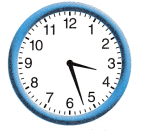3:52 P.M

Explanation:
Total time has taken 25 minutes.
So the ending time is 25+ 3:27 P.M = 3:52 P.M

Name the solid figure. Then write the number of faces, edges, and vertices. (TEKS 3.6.A)

Question 5.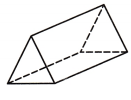__________ faces
__________ edges
__________ vertices
Triangular prism

Explanation:
5 faces
9 edges
6 vertices

Question 6.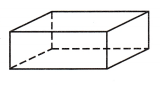__________ faces
__________ edges
__________ vertices
Rectangular Prism

Explanation:
6 faces
12 edges
8 vertices

Fill in the bubble for the correct answer choice.

Question 7.
Blake divided the figure at the right into parts with equal area. Which fraction names the area of each part of the divided figure? (TEKS 3.6.E)(A) $$\frac{1}{6}$$
(B) $$\frac{1}{8}$$
(C) $$\frac{1}{4}$$
(D) $$\frac{1}{3}$$
$$\frac{1}{3}$$

Explanation:
The fraction $$\frac{1}{3}$$ names the area of each part of the divided figure.

Question 8.
What is the area of the figure at the right? Each unit square is 1 square foot. (TEKS 3.6.D)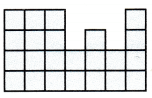(A) 28 square feet
(B) 23 square feet
(C) 27 square feet
(D) 20 square feet
23 square feet

Explanation:
The area of the figure at the right is 23 square feet.

Question 9.
Valentina is pouring water into a freshwater fish tank. What customary unit would be best to measure the capacity of the tank? (TEKS 3.7.D)
(A) cup
(B) quart
(C) gallon
(D) pint
gallon

Explanation:
The customary unit that would be best to measure the capacity of the tank is a gallon.

Question 10.
The perimeter of the figure at the right is 24 centimeters. What is the length of side w? (TEKS 3.7.B)(A) 3 centimeters
(B) 21 centimeters
(C) 4 centimeters
(D) 2 centimeters
3 centimeters.

Explanation:
The length of side w is 3 centimeters.

Question 11.
Spencer drew quadrilaterals with 2 pairs of sides of equal length. Which figure does NOT belong? (TEKS 3.6.B)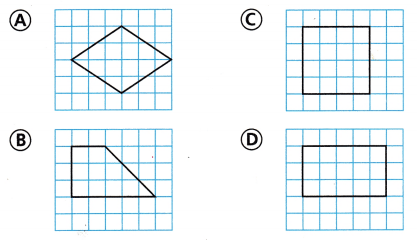Option B

Explanation:
The figure that does not belong is option B.

Fill in the bubble for the correct answer choice.

Question 12.
Jasmine is making a smoothie with the fruits at the right. How can she put the fruits in order from least to(A) watermelon, banana, strawberry
(B) banana, strawberry, watermelon
(C) strawberry, banana, watermelon
(D) Not here
Strawberry, banana, watermelon.

Explanation:
she put the fruits in order as Strawberry, banana, watermelon.

Question 13.
Which fraction shown names the point closest to 0 on the number line? (TEKS 3.7.A)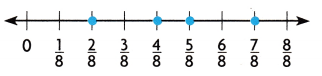(A) $$\frac{7}{8}$$
(B) $$\frac{2}{8}$$
(C) $$\frac{4}{8}$$
(D) $$\frac{5}{8}$$
$$\frac{2}{8}$$

Explanation:
The fraction shown names the point closest to 0 on the number line is $$\frac{2}{8}$$.

Question 14.
Bella is putting a puzzle together. She started at 2:20 P.M. and finished 35 minutes later. At what time did Bella finish the puzzle? (TEKS 3.7.C)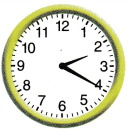(A) 1:45 P.M.
(B) 2:50 P.M.
(C) 2:35 P.M.
(D) 2:55 P.M.
2:55 P.M

Explanation:
The time Bella finishes the puzzle is 2:20+35 = 2:55 P.M.

Question 15.
A rectangular garden has a width of 6 feet and a perimeter of 32 feet. What is the length? (TEKS 3.7.B)
(A) 26 feet
(B) 10 feet
(C) 20 feet
(D) 38 feet
10 feet

Explanation:
Width = 6 feet
Perimeter = 32 feet
Perimeter = 2(l+b)
32 = 2(l+6)
32÷2 = l+6
16=l+6
l=16-6
l=10
The lenght is 10 feet.

Fill in the bubble for the correct answer choice.

Question 16.
Liam is sorting three-dimensional figures into those that have curved surfaces. Which figure would NOT be included? (TEKS 3.6.A)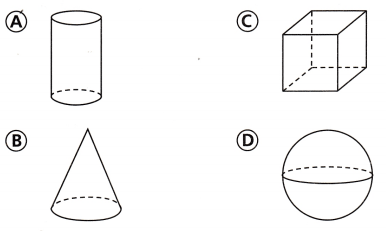Option c

Explanation:
Figure C is not included.

Question 17.
Mr. Weber designs houses. He is using grid paper to plan a new house design. The kitchen will have an area between 70 square feet and 85 square feet. The pantry will have an area between 4 square feet and 15 square feet.
Draw and label a diagram to show what Mr. Weber could design. Find the area of the kitchen. Find the area of the pantry.
Then find the total area of the kitchen and pantry combined. (TEKS 3.6.D )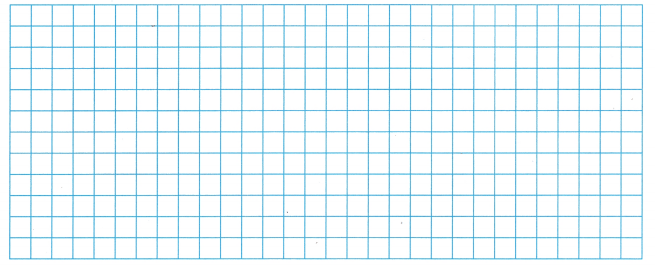Area of kitchen = __________ square feet
Area of pantry = __________ square feet
Total area of kitchen and pantry combined
= __________ square feet